Home » The Hackathon Practice Guide by Analytics Vidhya

# The Hackathon Practice Guide by Analytics Vidhya

## Introduction

A hackathon is a platform where you get the chance to apply your data science and machine learnin knowledge and techniques. It is a place where you can evaluate yourself by competing against, and learning from, fellow data science experts.

Here is an exclusive guide to help you prepare for participating in hackathons. This guide illustrates the list of important techniques which you should practice before stepping into the playing ground.

We’ll keep building this guide into a one place exhaustive resource for data science techniques and algorithms.

### 1. Framework of the Model Building Process

This is how the framework for model building works – you get data from multiple sources and then you perform the extraction and transformation operations. Once your data has been transformed, you apply your knowledge of predictive modeling and business understanding to build predictive models.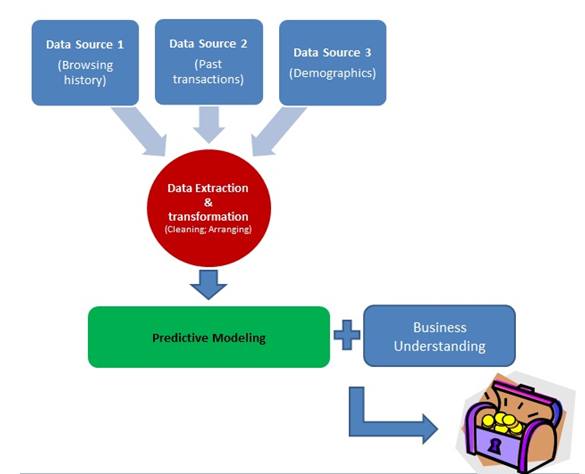### 2. Hypothesis Generation

• In your groups, list down all possible variables which might influence the independent variable (the variable to be predicted)
• Next, look at the dataset and see which variables are available

Make sure you always do this in this order

### 3.  Data Exploration and Feature Engineering

• Import the data set
• Variable identification
• Univariate, Bivariate and Multivariate analysis
• Identify and Treat missing and outlier values
• Create new variables or transform existing variables

Guides:

## Modelling Techniques

### 1) Logistic Regression

• Logistic regression is a form of regression analysis in which the outcome variable is binary or dichotomous
• Used when the focus on whether or not an event occurred, rather than when it occurred
• Here, instead of modelling the outcome Y directly, the method models the log odds(Y) using the logistic function
• Analysis of variance (ANOVA) and logistic regression all are special cases of General Linear Model (GLM)
• The probability of success falls between 0 and 1 for all possible values of X

#### a) Logit Transformation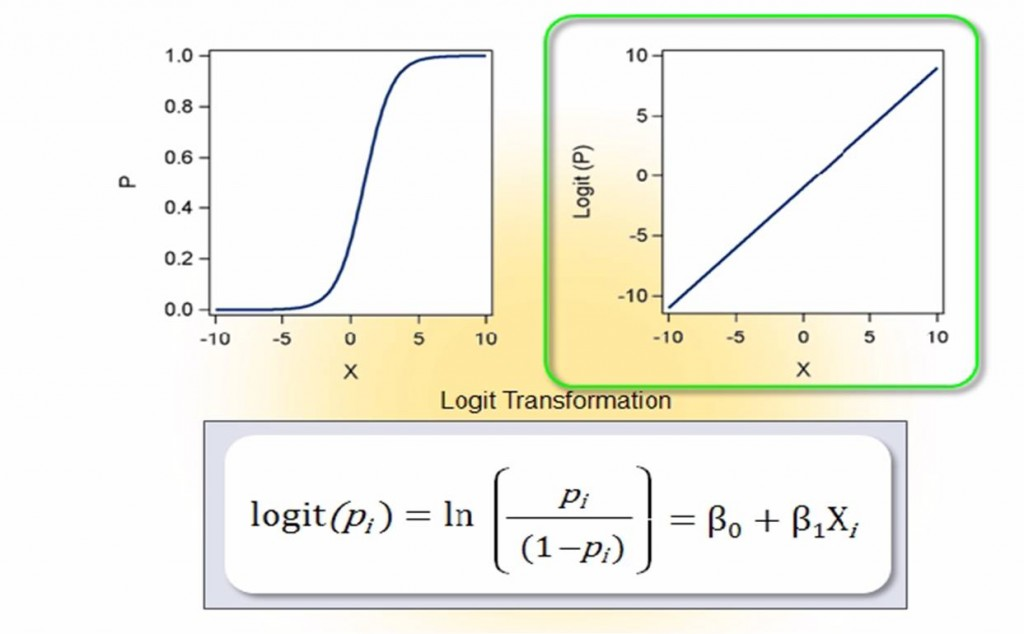b) Logit is directly related to Odds

• The logistic model can be written as: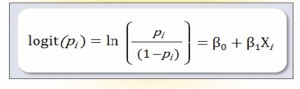• This implies that the odds for success can be expressed as: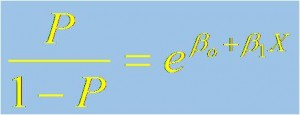• This relationship is the key to interpreting the coefficients in a logistic regression model

Guides:

### 2) Decision Tree

• Decision tree is a type of supervised learning algorithm
• It works for both categorical and continuous input and output variables
• It is a classification technique that split the population or sample into two or more homogeneous sets (or sub-populations) based on most significant splitter / differentiator in input variables

Decision Tree – Example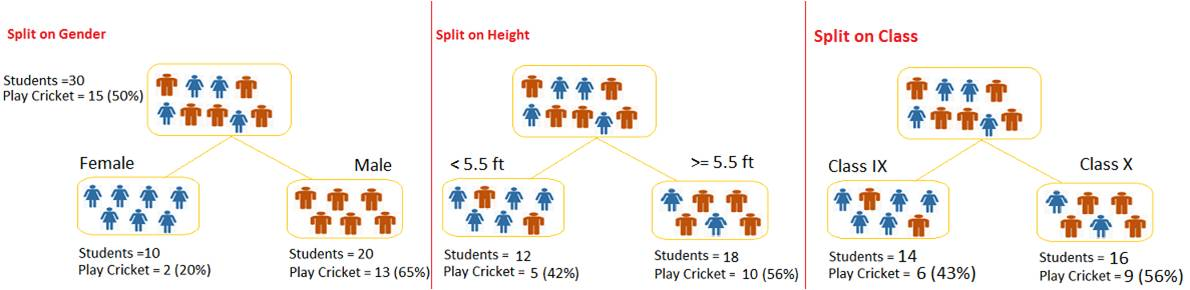Types of Decision Trees

• Binary Variable Decision Tree: Decision Tree which has binary target variable then it called as Binary Variable Decision Tree. Example:- In above scenario of student problem, where the target variable was “Student will play cricket or not” i.e. YES or NO.
• Continuous Variable Decision Tree: Decision Tree has continuous target variable then it is called as Continuous Variable Decision Tree.

Decision Tree – Terminology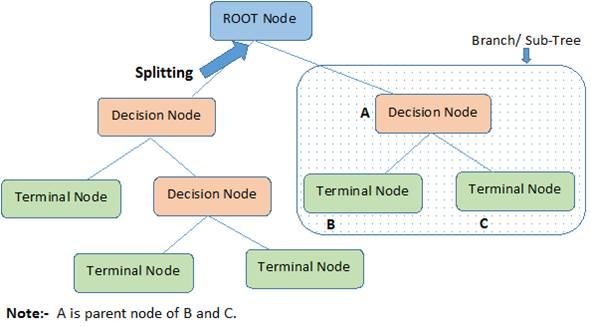• Easy to understand
• Useful in data exploration
• Less Data Cleaning required
• Data type is not a constraint

• Overfit
• Not fit for continuous variables
• Not Sensitive to Skewed distributions

Guides:

### 3) Random Forest

• “Random Forest“ is an algorithm to perform very intensive calculations.
• Random forest is like a bootstrapping algorithm with Decision tree (CART) model.
• Random forest gives much more accurate predictions when compared to simple CART/CHAID or regression models in many scenarios.
• It captures the variance of several input variables at the same time and enables high number of observations to participate in the prediction.
• A different subset of the training data and subset of variables are selected for each tree
• Remaining training data are used to estimate error and variable importance

• No need for pruning trees
• Accuracy and variable importance generated automatically
• Not very sensitive to outliers in training data
• Easy to set parameters

• Over fitting is not a problem
• It is black box, rules behind model building can not be explained

Guides:

### 4) Support Vector Machine(SVM)

• It is a classification technique.
• Support Vectors are simply the coordinates of individual observation
• Support Vector Machine is a frontier which best segregates the one class from other
• Solving SVMs is a quadratic programming problem
• Seen by many as the most successful current text classification method

### Case Study 1

We have a population of 50% males and 50% females. Here, we want to create some set of rules which will guide the gender class for the rest of the population.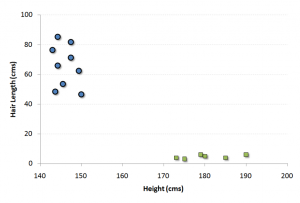The blue circles in the plot represent females and the green squares represents male.

Males in our population have a higher average height.

Females in our population have longer scalp hairs.

### Case Study 2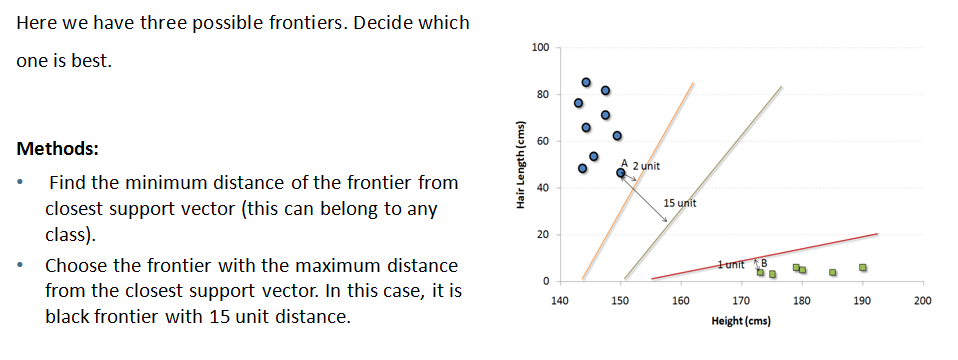### Text Mining:

Text mining is the analysis of data contained in natural language text. Text mining works by transposing words and phrases of unstructured data into numerical values which can then be linked with structured data in a database and analyzed with traditional data mining techniques.

Guides:

## End Notes

In this guide we talked about various modelling techniques, text analytics and the various stages which are necessary for a perfect model building.

### If you like what you just read & want to continue your analytics learning, subscribe to our emails, follow us on twitter or like our facebook page.

You can also read this article on our Mobile APP•Stella James says:

•RAKESH KUMAR says: